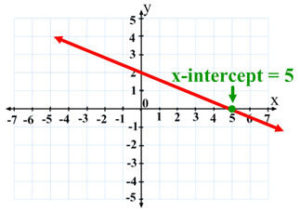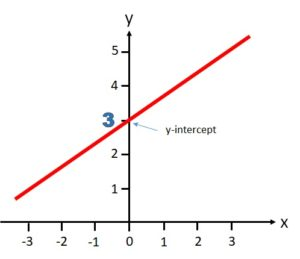# Find Intercepts: Examples of X, Y

## X-Intercept (Definition)

The x-intercept is the point where the graph of the plotted line crosses the x-axis.  On the graph below, the plotted line crosses the x-axis at x = 5.Eyeballing the graph isn’t an exact method. For example, in the graph above, the actual x-intercept could fall somewhere between 4.9 and 5.1. A more precise method for finding the intercept is to use the equation for a line.  The universal formula for every straight line, a linear equation, is:

y = mx + b

Where:

• m = the slope of the line (its steepness).  The slope is found by dividing the change in y-value by the change in x-value,
• b = the y-intercept,
• x = the x-value.

You will often need to find the value of the x, given values for m and b. For that, you’ll need to use a little algebra.

## Example: Finding the X-Intercept

Example question: What is the x-intercept of the equation y = 3x – 1?

Step 1: Set y to O.

0 = 3x – 1

Step 2: Add 1 to each side:

0 (+ 1) = 3x – 1 (+ 1)
1 = 3x

Step 3: Divide both sides by 3:

1 (/3) = 3x (/3)
⅓ = x

The x-intercept for the above equation is 1/3.

Another case where you will come across the x-intercept is in dealing with quadratic functions.  A quadratic equation has two solutions; The line is in the form of a parabola, which means that there will be two x-intercepts.  The standard quadratic equation is:

y = ax2 + bx = c

In this example, you’ll need to use the quadratic formula to find the x-intercept.

## Y-Intercept (Definition)

The y-intercept is the point where a graph crosses the y-axis.  On the graph below, the plotted line crosses the y-axis at y = 3:## More Examples

To find the y-intercept on a graph, just look for the place where the line crosses the y-axis (the vertical line).Note that you can have more than one y intercept, as in the third picture, which has two y intercepts. In calculus, you won’t see more than one y intercept, because it creates a major issue called “one to many.” In other words, your graph fails to represent a function (it might be a valid equation, but in calculus, you have to have functions in order for the math to work!).

## Y-Intercepts in Linear Equations

The universal formula for every straight line, a linear equation, is:

y = mx + b

Where:

• m is the slope of the line (its steepness).
• b is the y-intercept.

Sometimes, the intercept can be found just by looking at the value in the b position. For example, in the linear equation y = 2x – 2, the line will intersect the y-axis at -2.You will often need to calculate the value of b, however, given values for m and x.

## Example: Finding the Y-Intercept

Example question: At what point on the y-axis will a line with a coordinate point (1,1000) and a slope of 750 pass?

Step 1: Insert each of the given numbers into the standard linear equation:

1. y = mx + b
2. 1000 = 750(1) + b
3. 1000 = 750 + b

Step 2: Solve for b using algebra.

1. Subtract 750 from both sides: 1000 (-750) = 750 (-750) + b
2. 250 = b

The y-intercept of the line is 250.  The plotted line will pass through the y-axis at point 250.

## Y-Intercept in a Quadratic Equation

Another case where you will encounter y-intercept is in dealing with quadratic equations.  In a standard quadratic equation:

y = ax2 + bx + c

The intercept is represented by point c.  In the following equation:

y = 2x – x + 4

the y-intercept is 4.

## How To Find Intercepts

Intercepts are where the function crosses the x-axis (the x-intercept) and the y-axis (the y-intercept). There are several ways you can find intercepts:

• The guess and check method,
• Factoring,
• Finding the solution using a graphing calculator.

Guess and check works well for very simple equations like y = x + 2, but you’ll rarely be dealing with simple equations in calculus. Most graphing calculators have the ability to solve for intercepts (you can find a simple online calculator here). However, in many cases (especially in elementary algebra or pre-calculus), you’ll need to find the solutions algebraically. If you don’t have strong algebra skills, you might run into difficulties. However, referring to a graph can help, so can calculators like this one (which factors for you).

## How to Find Intercepts in Calculus: Factoring Example

Example problem 1: Find the intercepts of the function

• y = x3 – 9x.Step 1: Find the x-intercept(s):

1. Set the function equal to zero: x3 – 9x = 0
2. Factor: x(x – 3) (x + 3) = 0,
3. so x = 0, x = -3, x = 3.

Written as ordered pairs, the x-intercepts are (-3, 0), (0, 0) and (3, 0).

Step 2: Solve to find the y-intercept:

1. x3 – 9x = y
2. (0)3 – 9(0) = y
3. y = 0

## How to Find Intercepts in Calculus: Quadratic Formula Example

Step 1: Set x to 0 in your function to find the x-intercept:
02 – 2(0) -1 = -1.
Written as an ordered pair, the x-intercept is (0, -1).

Step 2. Set y to 0 and then solve to find the y-intercept:

1. 0 = x2 – 2x -1 (setting y to zero)
2. x = -1± √ 2 (using the quadratic formula to solve)
3. Therefore, there are two intercepts at (-1- √ 2, 0) and (-1 + √ 2, 0).

Tip: Check your solutions on a graphing calculator if you can, to see if they make sense. Looking at this particular graph, we can clearly see that there are two points where the graph crosses the x-axis and one point where it crosses the y = axis.

## References

Stewart et al. (2003). College Algebra. Cengage Learning.

CITE THIS AS:
Stephanie Glen. "Find Intercepts: Examples of X, Y" From StatisticsHowTo.com: Elementary Statistics for the rest of us! https://www.statisticshowto.com/calculus-problem-solving/find-intercepts-x-y/